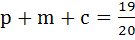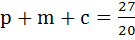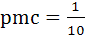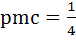Question 22

The probability that a student passes in Mathematics, Physics and Chemistry are m, p and c respectively. Of these subjects, the student has 75% chances of passing in at least one, a 50% chance of passing in at least two and 40% chance of passing in exactly two. Which of the following relations is true?

a)b)c)d)IIT 1998
 Your Answer ABCD CLEAR « Previous Answer: b, c Next »